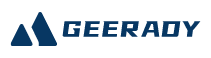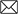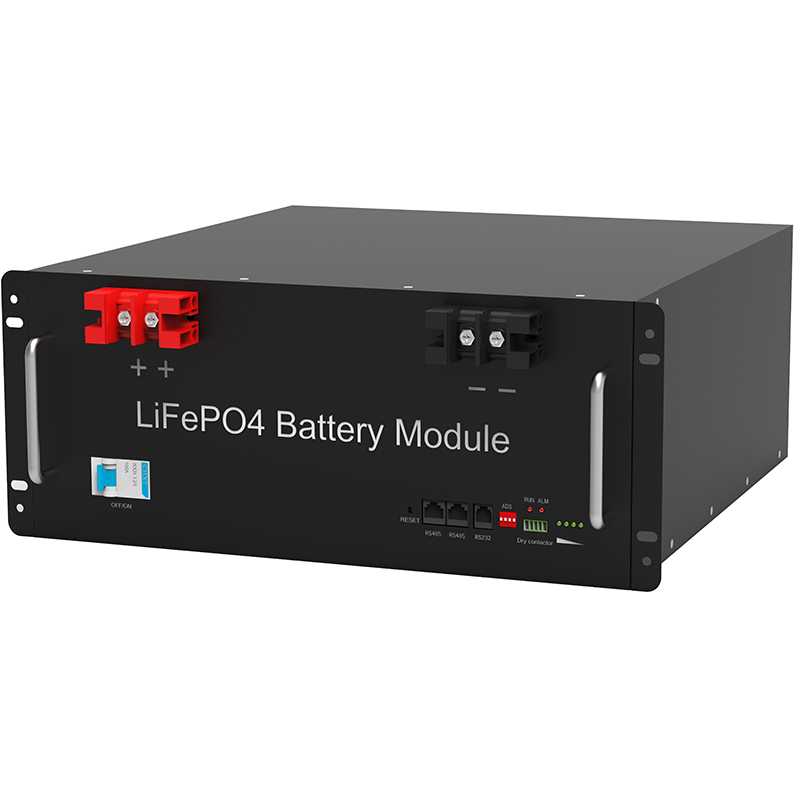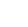#Emial:sales@geerady.com
Location： > > Opening voltage and capacity notes for lead-acid batteries

### Opening voltage and capacity notes for lead-acid batteries

Return Listsource：Gratene date： 2022-06-17The electrical energy of the lead-acid battery uses the following parameters: battery electromotive, open circuit voltage, termination voltage, operating voltage, discharge current, capacity, battery internal resistance, storage performance, service life (floating service life, charge and discharge cycle life).

1, battery electromotive, open circuit voltage, operating voltage
Be
When the battery conductor is turned on, the electrochemical reaction of the positive electrode and the negative electrode is carried out spontaneously. If the electrical energy and chemical energy conversion in the battery reach a balance, the positive electrode balanced electrode potential is the difference of the electrode potential. It is equivalent to the open circuit voltage when it is equal to the steady value. The product of the electric potential and the unit power indicates the maximum power of the unit power. However, the battery’s electric heat and open circuit voltage is different: the electromotive force can be based on the reaction of the reaction using thermodynamic calculations or by measurement, there is a clear physical significance. The latter is only near the electric potential on the number, and it is necessary to depend on the reach of the battery.
Be
The battery voltage in the open state is referred to as an open circuit voltage. The open circuit voltage of the battery is equal to the difference between the positive electrode electrode potential of the battery and the negative electrode electrode.
Be
Battery operating voltage refers to a battery having a current through (closed circuit). The starting voltage of the battery discharge is called an initial voltage. After the battery is on, the battery’s operating voltage is lower than the open circuit voltage due to the presence of ohmic resistance and polarization over-potential.
Be
2, capacity
Be
Battery capacity refers to the number of battery storage power, indicated by symbol C. Commonly used units are ampere hours, referred to as safety (AH) or mAh (MAH).
Be
The capacity of the battery can be divided into rated capacity (nominal capacity), actual capacity.
Be
(1) Rated capacity
Be
The rated capacity is that the battery specifies at a temperature of 25 ° C, which is discharged at a 10-hour current, and the minimum amount of electricity (AH) should be released.
Be
A, discharge rate. The discharge ratio is for the battery discharge current size, divided into time rate and current.
Be
The discharge time index refers to the length of time to discharge to the discharge of the discharge in a certain discharge condition. Depending on the IEC standard, the discharge time rate has 20, 10, 5, 3, 1, 0.5 hours and minute ratio, respectively, respectively, 20Hr, 10Hr, 5Hr, 3Hr, 2Hr, 1Hr, 0.5HR, etc.
Be
B. Discharge Termination Voltage. The lead battery is discharged at a ambient temperature of 25 ° C at a ambient temperature of 25 ° C to refer to the low voltage, referred to as discharge. Most fixed batteries specify that the voltage is 1.8V / only at 10 HR discharge (25 ° C). Terminate the voltage value and needs to be confined. Typically, in order to operate safely, small current discharges of less than 10 hr, the termination voltage is slightly higher, greater than 10 hr, and the termination voltage is slightly lower. In the communication power system, the termination voltage of the battery discharge is determined by the communication device to the base voltage.
Be
The discharge current rate is established to compare the battery discharge current sizes of different nominal capacity, usually in a 10-hour current, indicated by I10, 3 hours and 1 hour discharge current, respectively, respectively, respectively, respectively, respectively.
Be
C, rated capacity. The fixed lead-acid battery specifies the rated capacity that is discharged to the final voltage in a 10-hour current in the 25 ° C in an environment. The 10-hour rated capacity is represented by C10. The 10-hour current value is
Be
The method of the other hour rate is:
Be
The 3-hour rate capacity (AH) is represented by C3, and the measured capacity (AH) at ambient temperature at 25 ° C is the product of the discharge current and the discharge time (H), and the valve-controlled lead-acid fixed battery C3 and I3 value should be
Be
C3 = 0.75c10 (AH)
Be
I3 = 2.5i10 (h)
Be
1 hourly capacity (AH) is represented by C1, the measured C1 and I1 value should be
Be
C1 = 0.55c10 (AH)
Be
I1 = 5.5i10 (h)
Be
(2) actual capacity
Be
The actual capacity refers to the amount of electricity that the battery can output under certain conditions. It is equal to the product of discharge current and discharge time, in AH.
Be
3, internal resistance
Be
The internal resistance of the battery includes ohmic internal resistance and polarization internal resistance, and the polarized internal resistance includes electrochemical polarization and concentration. The presence of the internal resistance makes the end voltage at the time of the battery discharge than the battery electromotive force and the open circuit voltage, and the charging time voltage is higher than the electric potential and the open circuit voltage. The internal resistance of the battery is not constant, and continuously changes over time during the charge and discharge process, because the composition of the active material, the electrolyte concentration and temperature are constantly changing.
Be
Ohm resistors comply with Ohm’s law; the polarization resistance increases with current density, but is not linear relationship, and the logarithm of the current density increases.
Be
4, cycle life
Be
The battery experiences charge and discharge, called a cycle (one cycle). Under a certain discharge condition, the battery can withstand the number of cycles that the battery can withstand before the battery works to a certain capacity specified value, called a cycle life.
Be
The number of cycles of various batteries is different, and the conventional fixed lead-acid battery is about 500 to 600 times, and the start-type lead-acid battery is about 300 to 500 times. Valve-controlled sealing lead acid battery cycle life is 1000 ~ 1200 times. Factors affecting the life life First, the performance of the manufacturer products, the second is the quality of maintenance. The life of a fixed lead battery can also be measured with a floating life (year), and the valve-controlled lead-acid battery floating life is more than 10 years.
Be
For the start-type lead-acid battery, according to my country’s mechanical power department, the overcharge durability and the number of cyclic durability units are used to indicate the life, and the number of cycles is used to represent life. That is, the number of overcharge units should be 4 or more, and the number of cyclic durable capacity units should be 3 or more.
Be
5, energy
Be
The energy of the battery refers to the electrical energy given by the battery in a certain discharge system, usually expressed as a wattage (WH).
Be
The energy of the battery is divided into theoretical energy and actual energy. Theoretical energy w care the product of the theoretical capacity and the electric potential (E), namely
Be
W 理 = C 理 E
Be
The actual energy of the battery is the product of the actual capacity C in a certain discharge condition and the average operating voltage U flat product, ie
Be
W is = C real u flat
Be
Commonly used energy compared to compare different battery systems. Compared to energy refers to electrical energy output by battery unit or unit volume, the units are WH / KG or WH / L, respectively.
Be
It is more equivalent to energy and actual than energy than energy. The former refers to the energy that can be output in theory when the 1kg battery reaction material is completely discharged. The actual energy can be output than the energy of 1kg battery reaction material.
Be
Due to the influence of various factors, the actual amount of the battery is much smaller than the theoretical ratio energy. The actual relationship between energy and theoretical than energy can be represented as follows:
Be
W true = w 理 • KV • KR • KM
Be
KV-voltage efficiency in the formula; KR-reaction efficiency; KM-mass efficiency.
Be
Voltage efficiency refers to the ratio of the operating voltage of the battery and the electromotive force. When the battery is discharged, the operating voltage is less than the electric potential due to electrochemical polarization, concentration polarization and ohmic pressure drop.
Be
The reaction efficiency represents the utilization of the active substance.
Be
The specific energy of the battery is a comprehensive indicator, which reflects the quality level of the battery, which also shows the technical and management level of the manufacturer.
Be
6, storage performance
Be
The battery can form a microbial cell with a negative electrode active material, a negative electrode metal dissolution, and a precipitation of a negative electrode metal dissolution and hydrogen. In addition, impurities dissolved from the positive electrode plate grid, if the standard electrode potential is between the positive electrode and the negative electrode electrode potential, it is oxidized, and it is reduced by the negative electrode. Therefore, the presence of harmful impurities gradually consumes the positive and negative electrode active substances, and causes the battery loss capacity, which is called self-discharge.
Be
Battery self-discharge rate is reduced by a percentage of unit time content: i.e., the capacity of capacity difference values ​​and storage time t (days, month) before storage (C10 ‘) (day, month).

Recommend:

####TEL:

86-0755-36949293